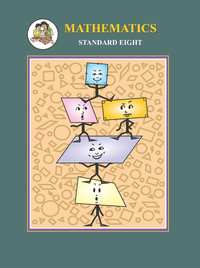Share

# Balbharati solutions for Maharashtra state board (SSC) Class 8 Mathematics chapter 14 - Compound interest [Latest edition]

Textbook page

#### Chapters## Chapter 14: Compound interest

Practice Set 14.1Practice Set 14.2

### Balbharati solutions for Maharashtra state board (SSC) Class 8 Mathematics Chapter 14 Compound interest Practice Set 14.1 [Page 90]

Practice Set 14.1 | Q 1 | Page 90

Find the amount and the compound interest.

 No. Principal (₹) Rate (p.c.p.a.) Duration(Years) 1 2000 5 2 2 5000 8 3 3 4000 7.5 2
Practice Set 14.1 | Q 2 | Page 90

Sameerrao has taken a loan of ₹12500 at a rate of 12 p.c.p.a. for 3 years. If the interest is compounded annually then how many rupees should he pay to clear his loan?

Practice Set 14.1 | Q 3 | Page 90

To start a business Shalaka has taken a loan of ₹8000 at a rate of 10 1/2 p.c.p.a. After two years how much compound interest will she have to pay?

### Balbharati solutions for Maharashtra state board (SSC) Class 8 Mathematics Chapter 14 Compound interest Practice Set 14.2 [Page 93]

Practice Set 14.2 | Q 1 | Page 93

On the construction work of a flyover bridge there were 320 workers initially. The number of workers were increased by 25% every year. Find the number of workers after 2 years.

Practice Set 14.2 | Q 2 | Page 93

A shepherd has 200 sheep with him. Find the number of sheeps with him after 3 years if the increase in number of sheeps is 8% every year.

Practice Set 14.2 | Q 3 | Page 93

In a forest there are 40,000 trees. Find the expected number of trees after 3 years if the objective is to increase the number at the rate 5% per year.

Practice Set 14.2 | Q 4 | Page 93

The cost price of a machine is 2,50,000. If the rate of depreciation is 10% per year find the depreciation in price of the machine after two years.

Practice Set 14.2 | Q 5 | Page 93

Find the compound interest if the amount of a certain principal after two years is ₹4036.80 at the rate of 16 p.c.p.a.

Practice Set 14.2 | Q 6 | Page 93

A loan of ₹15000 was taken on compound interest. If the rate of compound interest s 12 p.c.p.a. find the amount to settle the loan after 3 years.

Practice Set 14.2 | Q 7 | Page 93

A principal amounts to ₹13924 in 2 years by compound interest at 18 p.c.p.a. Find the principal.

Practice Set 14.2 | Q 8 | Page 93

The population of a suburb is 16000. Find the rate of increase in the population if the population after two years is 17640.

Practice Set 14.2 | Q 9 | Page 93

In how many years ₹700 will amount to ₹847 at a compound interest rate of 10 p.c.p.a.

Practice Set 14.2 | Q 10 | Page 93

Find the difference between simple interest and compound interest on ₹20000 at 8 p.c.p.a.

## Chapter 14: Compound interest

Practice Set 14.1Practice Set 14.2## Balbharati solutions for Maharashtra state board (SSC) Class 8 Mathematics chapter 14 - Compound interest

Balbharati solutions for Maharashtra state board (SSC) Class 8 Mathematics chapter 14 (Compound interest) include all questions with solution and detail explanation. This will clear students doubts about any question and improve application skills while preparing for board exams. The detailed, step-by-step solutions will help you understand the concepts better and clear your confusions, if any. Shaalaa.com has the Maharashtra State Board Maharashtra state board (SSC) Class 8 Mathematics solutions in a manner that help students grasp basic concepts better and faster.

Further, we at Shaalaa.com provide such solutions so that students can prepare for written exams. Balbharati textbook solutions can be a core help for self-study and acts as a perfect self-help guidance for students.

Concepts covered in Maharashtra state board (SSC) Class 8 Mathematics chapter 14 Compound interest are Concept of Compound Interest, Application of Formula for Compound Interest.

Using Balbharati Class 8 solutions Compound interest exercise by students are an easy way to prepare for the exams, as they involve solutions arranged chapter-wise also page wise. The questions involved in Balbharati Solutions are important questions that can be asked in the final exam. Maximum students of Maharashtra State Board Class 8 prefer Balbharati Textbook Solutions to score more in exam.

Get the free view of chapter 14 Compound interest Class 8 extra questions for Maharashtra state board (SSC) Class 8 Mathematics and can use Shaalaa.com to keep it handy for your exam preparation